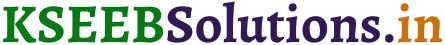# KSEEB Solutions for Class 7 Maths Chapter 13 Exponents and Powers Ex 13.3

Students can Download Class 7 Maths Chapter 13 Exponents and Powers Ex 13.3 Questions and Answers, Notes Pdf, KSEEB Solutions for Class 7 Maths helps you to revise the complete Karnataka State Board Syllabus and to clear all their doubts, score well in final exams.

## Karnataka State Syllabus Class 7 Maths Chapter 13 Exponents and Powers Ex 13.3

Question 1.
Write the following numbers in the expanded forms:
279404, 3006194, 2806196, 120719, 20068.
Solution:
279404 = 2 × 105 + 7 × 104 + 9 × 103 + 4 × 102 + 0 × 101 + 4 × 100
3006194 = 3 × 106 + 0 × 105 + 0 × 104 + 6 × 103 + 1 × 102 + 9 × 101 + 4 × 100
2806196 = 2 × 106 + 8 × 105 + 0 × 104 + 6 × 103+ 1 × 102 + 9 × 103 + 6 × 100
120719 = 1 × 105 + 2 × 104 + 0 × 103 + 7 × 102 + 1 × 101 + 9 × 100
20068 = 2 × 104 + 0 × 103 + 0 × 102 + 6 × 101 + 8 × 100Question 2.
Find the number from each of the following expanded forms:
a. 8 × 104 + 6 × 103 + 0 × 102 + 4 × 101 + 5 × 100
b. 4 × 105 + 5 × 103 + 3 × 102 + 2 × 100
c. 3 × 104 + 7 × 102 + 5 × 100
d. 9 × 105 + 2 × 102 + 3 × 101
Solution:
a. 86045
b. 405302
c. 30705
d. 900230

Question 3.
Express the following numbers in standard form:
(i) 5,00,00,000
(ii) 70,00,000
(iii) 3,18,65,00,000
(iv) 3,90,878
(v) 39087.8
(vi) 3908.78
Solution:
(i) 5,00,00,000 = 5 × 107
(ii) 70,00,000 = 7 × 106
(iii) 3,18,65,00,000 = 3.1865 × 109
(iv) 3,90,878 = 3.90878 × 105
(v) 39087.8 = 3.90878 × 104
(vi) 3908.78 = 3,90878 × 103Question 4.
Express the number appearing in the following statements in standard form.
(a) The distance between Earth and Moon is 384,000,000 m.
Solution:
3.84 × 108m

(b) Speed of light in vacuum is 300,000,000 m/s.
Solution:
3 × 108m/s

(c) Diameter of the Earth is 1,27,56,000 m.
Solution:
1.2756 × 107m

(d) Diameter of the Sun is 1,400,000,000 m.
Solution:
1.4 × 109m

(e) In a galaxy there are on an average 100,000,000,000 stars.
Solution:
1 × 1011

(f) The universe is estimated to be about 12,000,000,000 years old.
Solution:
1.2 × 1010 years.

(g) The distance of the Sun from the centre of the Milky Way Galaxy is estimated to be 300,000,000, 000,000,000,000 m.
Solution:
3 × 1020m.

(h) 60,230,000,000,000,000,000,000 molecules are contained in a drop of water weighing 1.8 gm.
Solution:
6.023 × 1022

(i) The earth has 1,353,000,000 cubic km of sea water.
Solution:
1.353 × 109 cubic km.

(j) The population of India was about 1,027,000,000 in March, 2001.
Solution:
1.027 × 109.